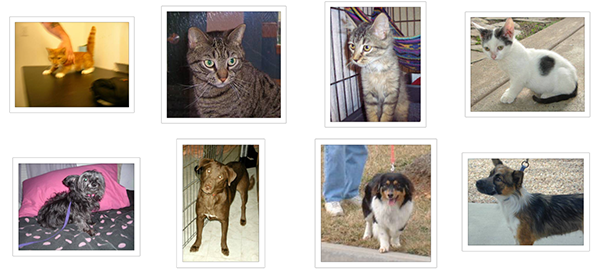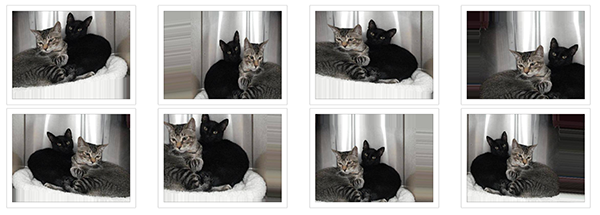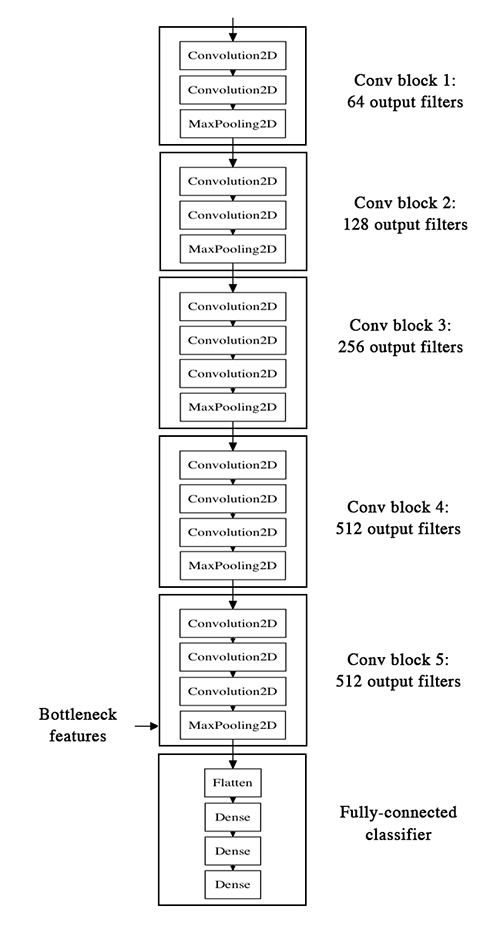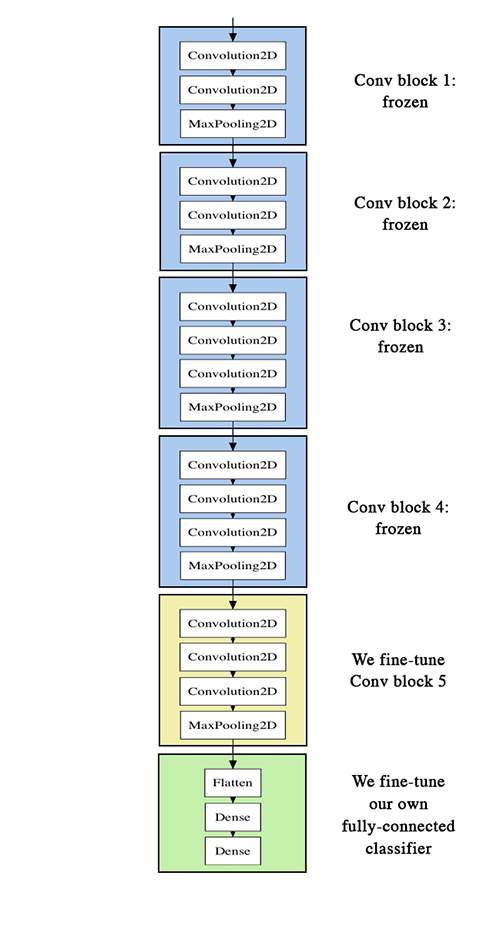# 面向小数据集构建图像分类模型

## 文章信息

### 概述

• 从图片中直接训练一个小网络（作为基准方法）

• 利用预训练网络的bottleneck（瓶颈）特征

• fine-tune预训练网络的高层

• `fit_generator`：用于从Python生成器中训练网络

• `ImageDataGenerator`：用于实时数据提升

• 层参数冻结和模型fine-tune

### 配置情况

• 2000张训练图片构成的数据集，一共两个类别，每类1000张

• 安装有Keras，SciPy，PIL的机器，如果有NVIDIA GPU那就更好了，但因为我们面对的是小数据集，没有也可以。

• 数据集按照下面的形式存放

``````data/
train/
dogs/
dog001.jpg
dog002.jpg
...
cats/
cat001/jpg
cat002.jpg
...
validation/
dogs/
dog001.jpg
dog002.jpg
...
cats/
cat001/jpg
cat002.jpg
...
``````### 数据预处理与数据提升

• 在训练过程中，设置要施行的随机变换

• 通过`.flow``.flow_from_directory(directory)`方法实例化一个针对图像batch的生成器，这些生成器可以被用作keras模型相关方法的输入，如`fit_generator``evaluate_generator``predict_generator`

``````from keras.preprocessing.image import ImageDataGenerator

datagen = ImageDataGenerator(
rotation_range=40,
width_shift_range=0.2,
height_shift_range=0.2,
rescale=1./255,
shear_range=0.2,
zoom_range=0.2,
horizontal_flip=True,
fill_mode='nearest')
``````

• `rotation_range`是一个0~180的度数，用来指定随机选择图片的角度。

• `width_shift``height_shift`用来指定水平和竖直方向随机移动的程度，这是两个0~1之间的比例。

• `rescale`值将在执行其他处理前乘到整个图像上，我们的图像在RGB通道都是0~255的整数，这样的操作可能使图像的值过高或过低，所以我们将这个值定为0~1之间的数。

• `shear_range`是用来进行剪切变换的程度，参考剪切变换

• `zoom_range`用来进行随机的放大

• `horizontal_flip`随机的对图片进行水平翻转，这个参数适用于水平翻转不影响图片语义的时候

• `fill_mode`用来指定当需要进行像素填充，如旋转，水平和竖直位移时，如何填充新出现的像素

``````from keras.preprocessing.image import ImageDataGenerator, array_to_img, img_to_array, load_img

datagen = ImageDataGenerator(
rotation_range=40,
width_shift_range=0.2,
height_shift_range=0.2,
shear_range=0.2,
zoom_range=0.2,
horizontal_flip=True,
fill_mode='nearest')

img = load_img('data/train/cats/cat.0.jpg')  # this is a PIL image
x = img_to_array(img)  # this is a Numpy array with shape (3, 150, 150)
x = x.reshape((1,) + x.shape)  # this is a Numpy array with shape (1, 3, 150, 150)

# the .flow() command below generates batches of randomly transformed images
# and saves the results to the `preview/` directory
i = 0
for batch in datagen.flow(x, batch_size=1,
save_to_dir='preview', save_prefix='cat', save_format='jpeg'):
i += 1
if i > 20:
break  # otherwise the generator would loop indefinitely
``````### 在小数据集上训练神经网络：40行代码达到80%的准确率

``````from keras.models import Sequential
from keras.layers import Convolution2D, MaxPooling2D
from keras.layers import Activation, Dropout, Flatten, Dense

model = Sequential()
model.add(Convolution2D(32, 3, 3, input_shape=(3, 150, 150)))

# the model so far outputs 3D feature maps (height, width, features)
``````

``````model.add(Flatten())  # this converts our 3D feature maps to 1D feature vectors

model.compile(loss='binary_crossentropy',
optimizer='rmsprop',
metrics=['accuracy'])
``````

``````# this is the augmentation configuration we will use for training
train_datagen = ImageDataGenerator(
rescale=1./255,
shear_range=0.2,
zoom_range=0.2,
horizontal_flip=True)

# this is the augmentation configuration we will use for testing:
# only rescaling
test_datagen = ImageDataGenerator(rescale=1./255)

# this is a generator that will read pictures found in
# subfolers of 'data/train', and indefinitely generate
# batches of augmented image data
train_generator = train_datagen.flow_from_directory(
'data/train',  # this is the target directory
target_size=(150, 150),  # all images will be resized to 150x150
batch_size=32,
class_mode='binary')  # since we use binary_crossentropy loss, we need binary labels

# this is a similar generator, for validation data
validation_generator = test_datagen.flow_from_directory(
'data/validation',
target_size=(150, 150),
batch_size=32,
class_mode='binary')
``````

``````model.fit_generator(
train_generator,
samples_per_epoch=2000,
nb_epoch=50,
validation_data=validation_generator,
nb_val_samples=800)
model.save_weights('first_try.h5')  # always save your weights after training or during training

``````

### 使用预训练网络的bottleneck特征：一分钟达到90%的正确率

VGG-16的网络结构如下：``````generator = datagen.flow_from_directory(
'data/train',
target_size=(150, 150),
batch_size=32,
class_mode=None,  # this means our generator will only yield batches of data, no labels
shuffle=False)  # our data will be in order, so all first 1000 images will be cats, then 1000 dogs
# the predict_generator method returns the output of a model, given
# a generator that yields batches of numpy data
bottleneck_features_train = model.predict_generator(generator, 2000)
# save the output as a Numpy array
np.save(open('bottleneck_features_train.npy', 'w'), bottleneck_features_train)

generator = datagen.flow_from_directory(
'data/validation',
target_size=(150, 150),
batch_size=32,
class_mode=None,
shuffle=False)
bottleneck_features_validation = model.predict_generator(generator, 800)
np.save(open('bottleneck_features_validation.npy', 'w'), bottleneck_features_validation)
``````

``````train_data = np.load(open('bottleneck_features_train.npy'))
# the features were saved in order, so recreating the labels is easy
train_labels = np.array( * 1000 +  * 1000)

validation_labels = np.array( * 400 +  * 400)

model = Sequential()

model.compile(optimizer='rmsprop',
loss='binary_crossentropy',
metrics=['accuracy'])

model.fit(train_data, train_labels,
nb_epoch=50, batch_size=32,
validation_data=(validation_data, validation_labels))
model.save_weights('bottleneck_fc_model.h5')
``````

### 在预训练的网络上fine-tune

• 搭建vgg-16并载入权重

• 将之前定义的全连接网络加在模型的顶部，并载入权重

• 冻结vgg16网络的一部分参数• 为了进行fine-tune,所有的层都应该以训练好的权重为初始值，例如，你不能将随机初始的全连接放在预训练的卷积层之上，这是因为由随机权重产生的大梯度将会破坏卷积层预训练的权重。在我们的情形中，这就是为什么我们首先训练顶层分类器，然后再基于它进行fine-tune的原因

• 我们选择只fine-tune最后的卷积块，而不是整个网络，这是为了防止过拟合。整个网络具有巨大的熵容量，因此具有很高的过拟合倾向。由底层卷积模块学习到的特征更加一般，更加不具有抽象性，因此我们要保持前两个卷积块（学习一般特征）不动，只fine-tune后面的卷积块（学习特别的特征）。

• fine-tune应该在很低的学习率下进行，通常使用SGD优化而不是其他自适应学习率的优化算法，如RMSProp。这是为了保证更新的幅度保持在较低的程度，以免毁坏预训练的特征。

``````# build a classifier model to put on top of the convolutional model
top_model = Sequential()

# classifier, including the top classifier,
# in order to successfully do fine-tuning

# add the model on top of the convolutional base
``````

``````# set the first 25 layers (up to the last conv block)
# to non-trainable (weights will not be updated)
for layer in model.layers[:25]:
layer.trainable = False

# compile the model with a SGD/momentum optimizer
# and a very slow learning rate.
model.compile(loss='binary_crossentropy',
optimizer=optimizers.SGD(lr=1e-4, momentum=0.9),
metrics=['accuracy'])
``````

``````# prepare data augmentation configuration
train_datagen = ImageDataGenerator(
rescale=1./255,
shear_range=0.2,
zoom_range=0.2,
horizontal_flip=True)

test_datagen = ImageDataGenerator(rescale=1./255)

train_generator = train_datagen.flow_from_directory(
train_data_dir,
target_size=(img_height, img_width),
batch_size=32,
class_mode='binary')

validation_generator = test_datagen.flow_from_directory(
validation_data_dir,
target_size=(img_height, img_width),
batch_size=32,
class_mode='binary')

# fine-tune the model
model.fit_generator(
train_generator,
samples_per_epoch=nb_train_samples,
nb_epoch=nb_epoch,
validation_data=validation_generator,
nb_val_samples=nb_validation_samples)
``````

• 更加强烈的数据提升

• 更加强烈的dropout

• 使用L1和L2正则项（也称为权重衰减）

• fine-tune更多的卷积块（配合更大的正则）